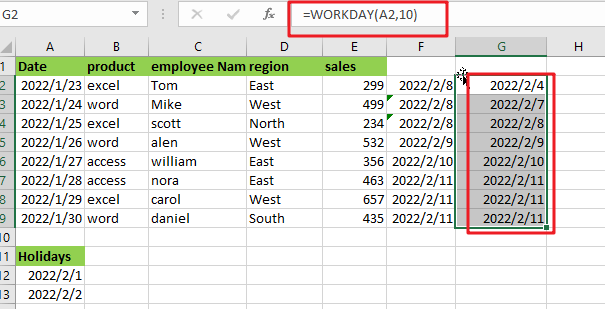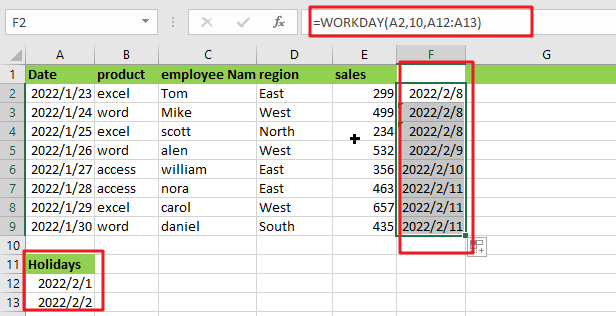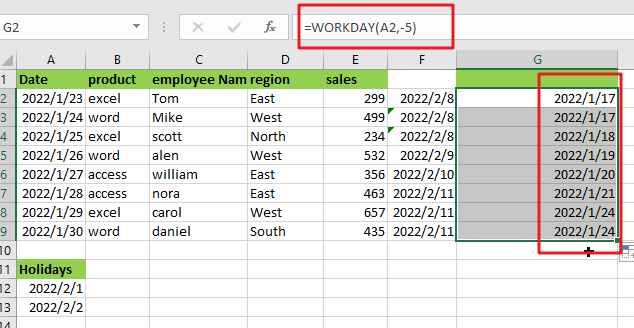You might have come across a situation where you need to add (or subtract) the specified business days to a particular date. There is also a possibility that you might have completed this kind of task manually. Still, it seems possible if you have to add or subtract specified days to a few dates, but when it comes to a lot of dates, then doing such tasks manually would be a foolish attempt because doing this task manually would not only exhaust you but also waste a lot of time, and as a result, your work would not complete on the specified time.

But don’t worry, since after attentively reading this post, you will learn the simplest way to add business days to a list of dates in a matter of seconds.

So without any delay, let’s get into it;## General Formula

In MS Excel, if you want to add days into a given date in just a matter of seconds, then  the following formula would help you out:

`=WORKDAY(start date,adding_days,holidays)`

## Explanation of Syntax:

Before getting to know the usage of this formula for getting the work done in an efficient way, we first need to understand each syntax so that you can understand how each syntax helps to add days into a given date in just a matter of seconds :

• `WORKDAY`: The WORKDAY function in MS Excel is used to compute the closest working day based on a value. Business days or workdays are any days other than Saturday and Sunday.
• `Start date`: This parameter provides the input dates.
• `Holidays`: This column indicates the additional holidays listed in the spreadsheet, so this formula reads this formula to decide if there is any holiday on those days.
• `Comma Symbol` (,): This symbol is like a separator that aids in the separation of a list of values.
• `Parenthesis` (): This primary function of this symbol is to group the elements.

## Explanation:

The formula WORKDAY function can subtract or add business days (workdays) from a date to generate a date in the past or future(respectively) that skips weekends (and holidays). Vacations are optional.

The formula in F2 in the example is:

`=WORKDAY(A2,10,A12:A13)`The above formula would add 10 days to dates in column A, and returns the results on column F.

Weekends are excluded by default from the WORKDAY function (Saturday and Sunday). In this example, we’ve also included a list of three holidays that occur within the date period being computed, implying that both weekends and holidays are eliminated.

## Subtract Business from a Date

You can provide a negative number for days to subtract business from a date (rather than adding workdays).

For example, to obtain a date five workdays before a date in A2, use:

` =WORKDAY(A2,-5)`If you find the above example challenging to grasp, don’t worry since another example below will walk you through the procedure step by step:

Take a look at the example image below.

1.  To begin, insert the input dates in Column A.
2. We’ll now compute the 10 working days minus the additional holiday specified in range .
3.  Enter the formula mentioned earlier into the formula bar section and press the ENTER:
` =WORKDAY(A2,10,A12:A13)`4. Finally, the result will be shown in the selected cell.

You can ignore the holidays range from the algorithm if you wish to compute the days without the supplied holidays. Please see the image below.

`=WORKDAY(A2,10)`## Conclusion

This blog post efficiently explains how you can use the basic Excel formula to add business days to a date that excludes weekends and holidays. I hope you understood it completely.

### Related Functions

• Excel WORKDAY function
The Excel WORKDAY function returns a serial number that represents a date that is the indicated number of working days before or after the starting date you specified.The syntax of the WORKDAY function is as below:=WORKDAY(start_date, days, [holidays])…